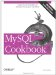# Calculating Linear Regressions or Correlation Coefficients

13.6.1 Problem

You want to calculate the least-squares regression line for two variables, or the correlation coefficient that expresses the strength of the relationship between them.

13.6.2 Solution

Apply summary functions to calculate the necessary terms.

13.6.3 Discussion

When the data values for two variables X and Y are stored in a database, the least-squares regression for them can be calculated easily using aggregate functions. The same is true for the correlation coefficient. The two calculations are actually fairly similar, and many terms for performing the computations are common to the two procedures.

Suppose you want to calculate a least-squares regression using the age and test score values for the observations in the testscore table:

```mysql> SELECT age, score FROM testscore;
+-----+-------+
| age | score |
+-----+-------+
| 5 | 5 |
| 5 | 4 |
| 5 | 6 |
| 5 | 7 |
| 6 | 8 |
| 6 | 9 |
| 6 | 4 |
| 6 | 6 |
| 7 | 8 |
| 7 | 6 |
| 7 | 9 |
| 7 | 7 |
| 8 | 9 |
| 8 | 6 |
| 8 | 7 |
| 8 | 10 |
| 9 | 9 |
| 9 | 7 |
| 9 | 10 |
| 9 | 9 |
+-----+-------+```

A regression line is expressed as follows, where a and b are the intercept and slope of the line:

`Y = bX + a`

Letting age be X and score be Y, begin by computing the terms needed for the correlation equation. These include the number of observations, the means, sums, and sums of squares for each variable, and the sum of the products of each variable:

 You can see where these terms come from by consulting any standard statistics text.

```mysql> SELECT
-> @n := COUNT(score) AS N,
-> @meanX := AVG(age) AS "X mean",
-> @sumX := SUM(age) AS "X sum",
-> @sumXX := SUM(age*age) "X sum of squares",
-> @meanY := AVG(score) AS "Y mean",
-> @sumY := SUM(score) AS "Y sum",
-> @sumYY := SUM(score*score) "Y sum of square",
-> @sumXY := SUM(age*score) AS "X*Y sum"
-> FROM testscoreG
*************************** 1. row ***************************
N: 20
X mean: 7.0000
X sum: 140
X sum of squares: 1020
Y mean: 7.3000
Y sum: 146
Y sum of square: 1130
X*Y sum: 1053```

From those terms, the regression slope and intercept are calculated as follows:

```mysql> SELECT
-> @b := (@n*@sumXY - @sumX*@sumY) / (@n*@sumXX - @sumX*@sumX)
-> AS slope;
+-------+
| slope |
+-------+
| 0.775 |
+-------+
mysql> SELECT @a :=
-> (@meanY - @b*@meanX)
-> AS intercept;
+-----------+
| intercept |
+-----------+
| 1.875 |
+-----------+```

The regression equation then is:

```mysql> SELECT CONCAT('Y = ',@b,'X + ',@a) AS 'least-squares regression';
+--------------------------+
| least-squares regression |
+--------------------------+
| Y = 0.775X + 1.875 |
+--------------------------+```

To compute the correlation coefficient, many of the same terms are used:

```mysql> SELECT
-> (@n*@sumXY - @sumX*@sumY)
-> / SQRT((@n*@sumXX - @sumX*@sumX) * (@n*@sumYY - @sumY*@sumY))
-> AS correlation;
+------------------+
| correlation |
+------------------+
| 0.61173620442199 |
+------------------+```MySQL Cookbook
ISBN: 059652708X
EAN: 2147483647
Year: 2005
Pages: 412
Authors: Paul DuBois